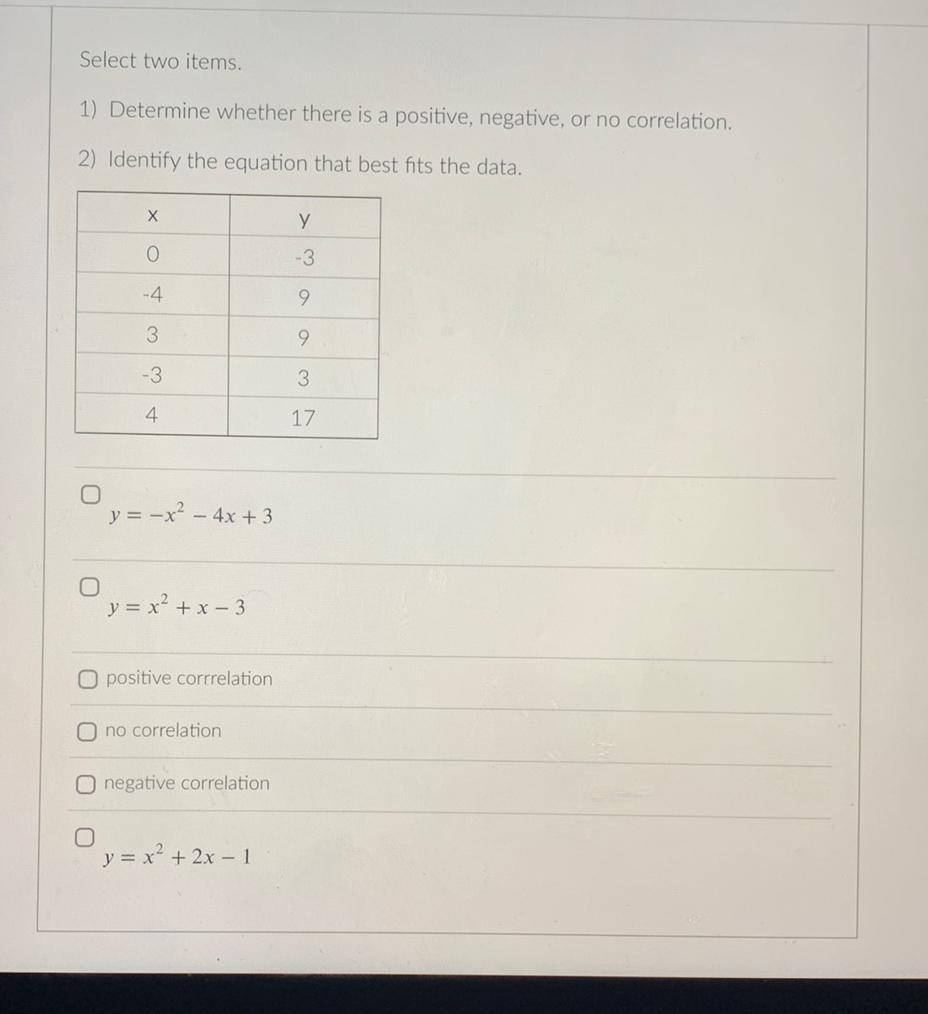Question:

# Select two items. 1) Determine whether there is a positive, negative, or no correlation. 2) Identify the equation that best fits the data. x y 0 -3 -4Select two items. 1) Determine whether there is a positive, negative, or no correlation. 2) Identify the equation that best fits the data. x y 0 -3 -4 9 3 9 -3 3 4 17 a)y = -x^2 - 4x + 3 b)y = x^2 + x - 3 c)positive corrrelation d)no correlation e)negative correlation f)y = x² + 2x - 1